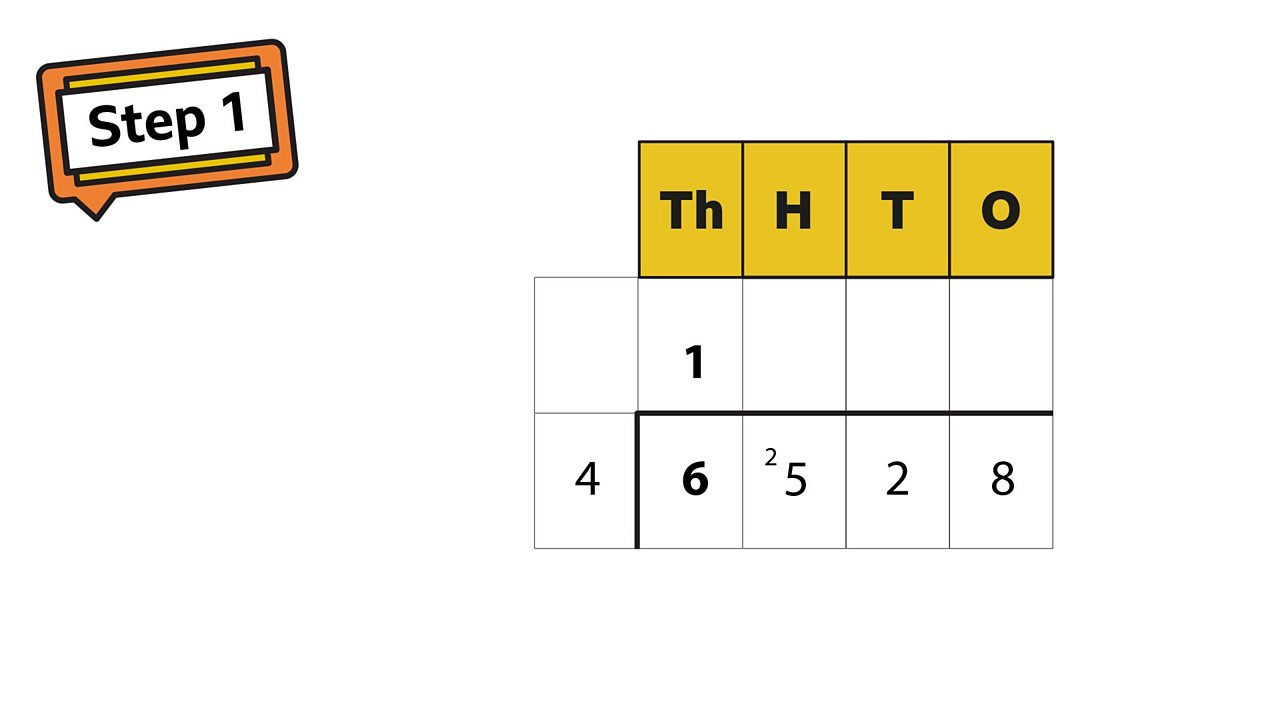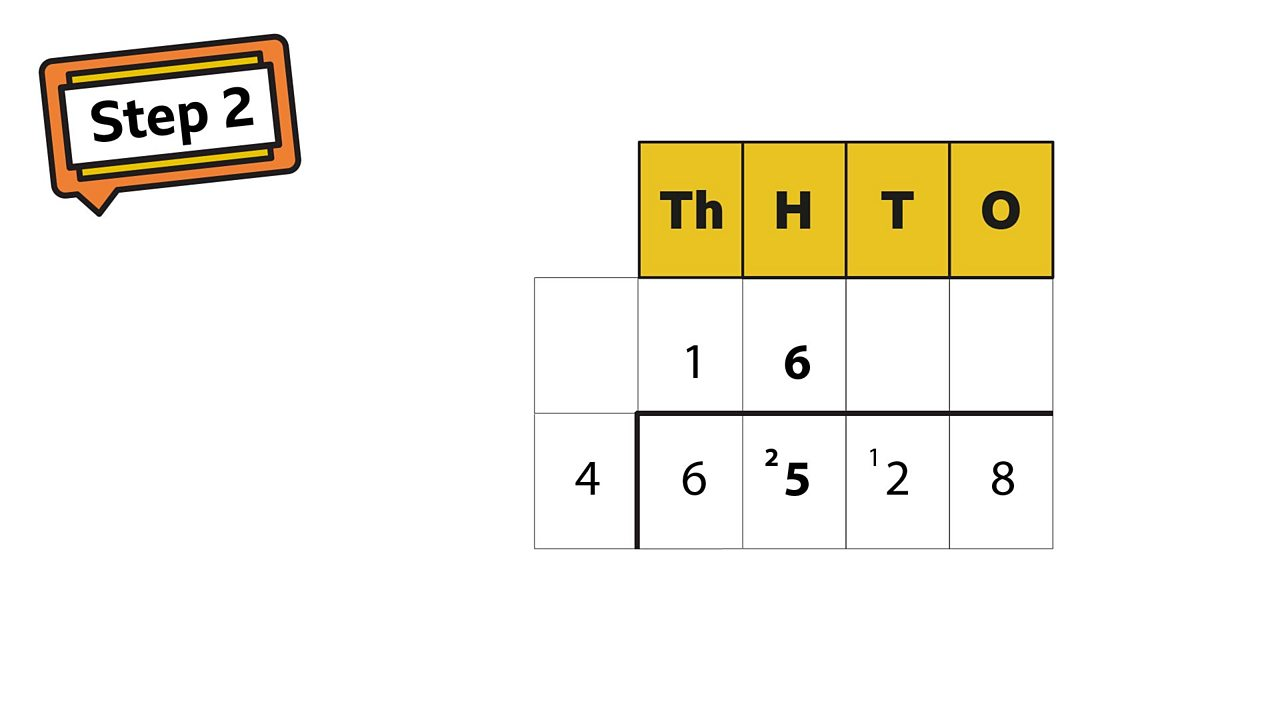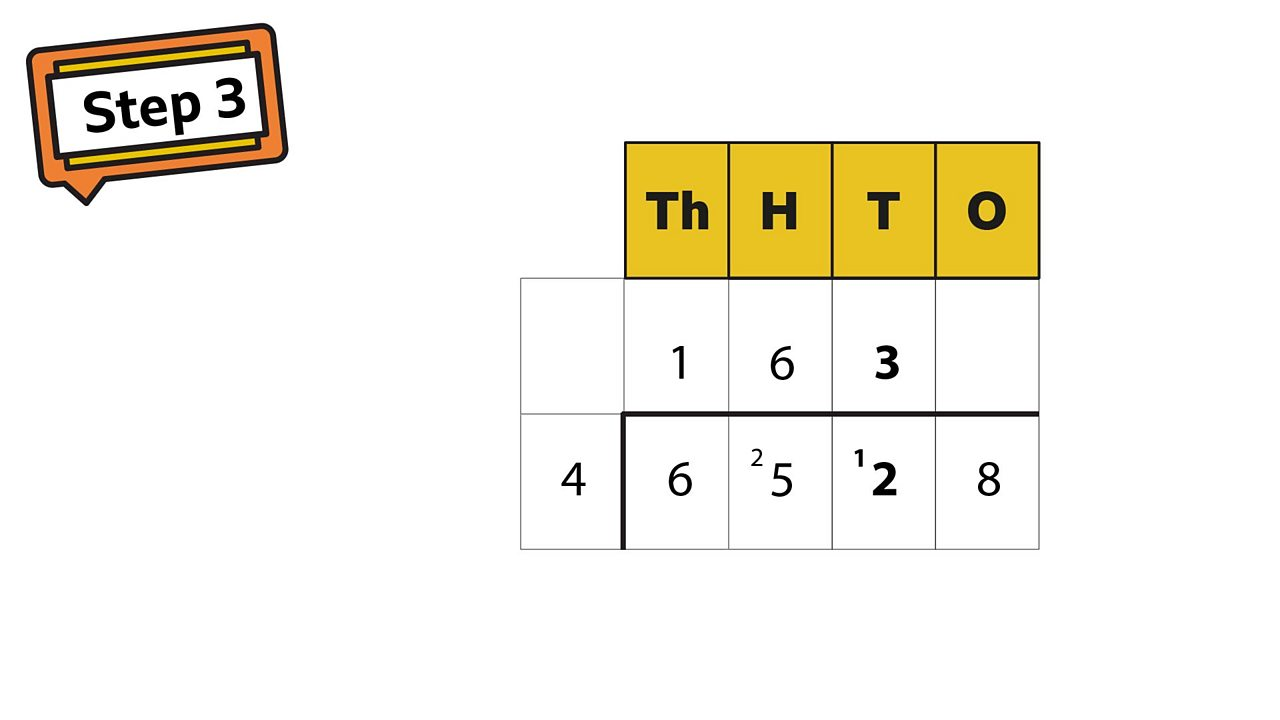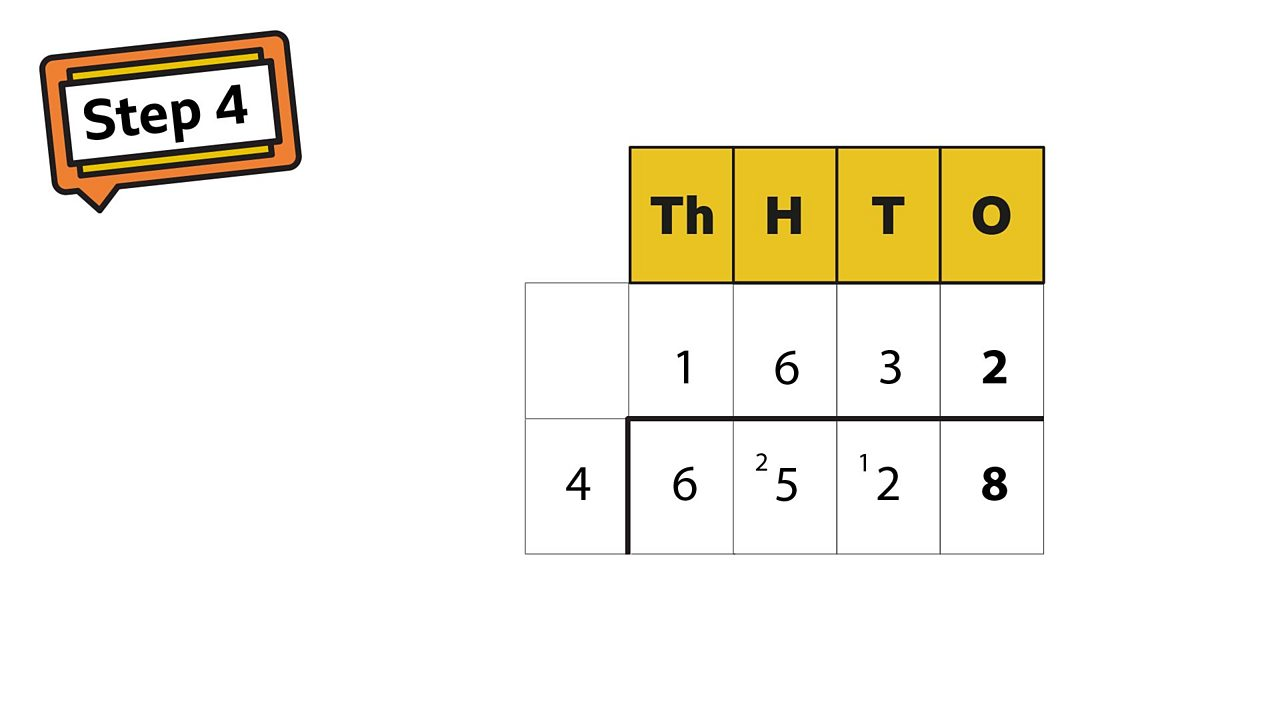# Divide up to 4-digits by 1-digit

## Learning focus

Learn how to do short division using written methods.

This lesson includes:

• a catch-up quiz
• a learning summary
• a videos
• two slideshows

# Quiz

Practise dividing up to 4-digits with this quiz.

# Learn

Watch this video to learn about using short division in a clothes shop.

## Short division

Short division will sometimes give an answer with a remainder (r), which you can round up or round down.

Short division is also known as the bus stop method because it looks like a bus stop. Once you understand the bus stop method, you can divide any number of digits by a 1-digit number.

## What is 6528 ÷ 4?4 into 6 is 1 remainder 2Start with the first digit in the number you are dividing into. In this case it is six thousands. How many 4s go into 6? 1 with 2 remainder. You carry the remainder over into the next place value, the hundreds.1 of 44 into 25 is 6 remainder 1How many fours go into 25? 25 ÷ 4 = 6 remainder 1. Carry the remainder into the tens.2 of 44 into 12 is 312 ÷ 4 = 3. No remainders here to carry over.3 of 44 into 8 is 2How many 4s in 8? 2. 6528 ÷ 4 = 16324 of 4

## Example 2

Sometimes there will be a remainder when you use the bus stop method.

# Practise

## Activity 1

Try this activity from Twinkl to practise short division. If you don't have a printer you can always make your own fish!

## Activity 2

Explore division further with this interactive lesson from My Maths, Oxford University Press.